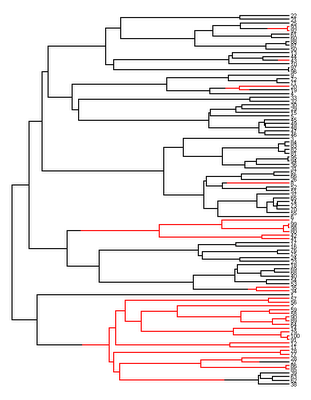## Tuesday, October 11, 2011

### Simulating a set number of changes in a discrete character on the tree using sim.history()

An R phylogenetics user recently queried as to whether it was possible to simulate random character evolution with a fixed number of changes - say 2, 5 or 10 - in a binary character on the tree. In fact, this can be done using the function sim.history() in the latest version of "phytools" (not yet available on CRAN, but downloadable from my website here).

I submitted the following code fragment:

nchanges<-5 # desired number of changes
ntrees<-100 # desired number of trees
ntaxa<-100 # desired number of taxa
require(phytools); require(geiger) # required packages
mtrees<-list()
for(i in 1:ntrees){
# simulate a stochastic B-D tree using birthdeath.tree
tree<-drop.tip(birthdeath.tree(b=1,d=0,taxa.stop=(ntaxa+1)),as.character(ntaxa+1))
# compute the rate that results in (on average) nchanges changes
q<-nchanges/sum(tree\$edge.length)
# simulate a stochastic history
mtree<-sim.history(tree,Q=matrix(c(-q,q,q,-q),2,2))
# loop until desired number of changes
while((length(unlist(mtree\$maps))-nrow(mtree\$edge))!=nchanges)
mtree<-sim.history(tree,Q=matrix(c(-q,q,q,-q),2,2))
mtrees[[i]]<-mtree
# for fun, plot the history
plotSimmap(mtrees[[i]])
}
class(mtrees)<-"multiPhylo"
# tip states for the kth tree are in mtrees[[k]]\$states

This is for a desired count of 5 changes on the tree. The way it works is by first computing the substitution rate that will produce an expected number of changes of 5 (in this case); and then by simulating repeatedly with this rate, retaining only those simulations that result in 5 changes on the tree.

The result is stored in a "simmap" style tree; with tip states in the list component \$states.

Below is an example of a tree simulated using this method, but with nchanges=10 and plotSimmap(...,pts=0,fsize=0.7):